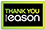# Numerical Methods and Data Analysis

PAPERBACK
Categories: Numerical analysis
In science and engineering, use of computers is indispensable. The computers are often used for numerical calculations of mathematical expressions. Use of the C and C++ languages is nowadays popular among the students of Science and Engineering. This book discusses numerical techniques: Interpolation, Differentiation, Integration, Roots of Equation, Simultaneous Linear Equations, Eigenvalues and Eigenvectors, Differential Equations, Partial Differential Equations, Random Numbers, Statistical Parameters, and the Error Analysis. For these techniques, the computer programs are written separately by using the C and C++ languages. One of the policies followed in this book has been to discuss each technique by solving an exercise with the help of calculator and then developing the computer program.
€54.53163 Reward Points
In stock online
Delivery in 2-3 working days

Any purchases for more than €10 are eligible for free delivery anywhere in the UK or Ireland!

In science and engineering, use of computers is indispensable. The computers are often used for numerical calculations of mathematical expressions. Use of the C and C++ languages is nowadays popular among the students of Science and Engineering. This book discusses numerical techniques: Interpolation, Differentiation, Integration, Roots of Equation, Simultaneous Linear Equations, Eigenvalues and Eigenvectors, Differential Equations, Partial Differential Equations, Random Numbers, Statistical Parameters, and the Error Analysis. For these techniques, the computer programs are written separately by using the C and C++ languages. One of the policies followed in this book has been to discuss each technique by solving an exercise with the help of calculator and then developing the computer program.
Quantity:
In stock online
Delivery in 2-3 working days163 Reward Points

Any purchases for more than €10 are eligible for free delivery anywhere in the UK or Ireland!

€54.53
In stock online
Delivery in 2-3 working days